Anzeige

# CS8392 OOP

23. Oct 2018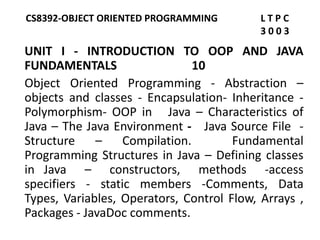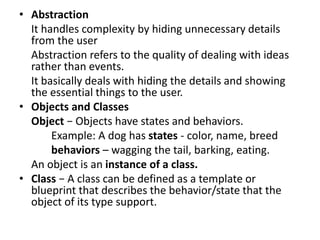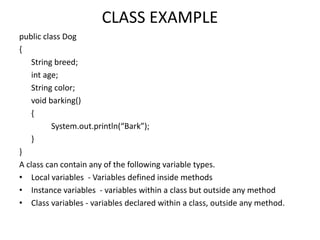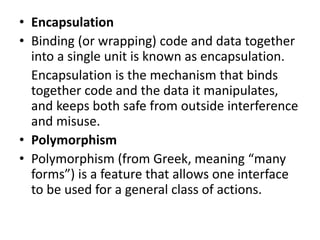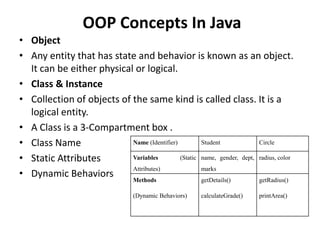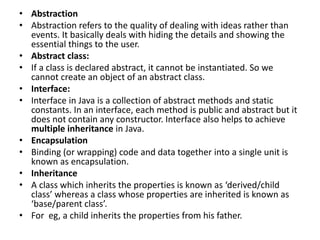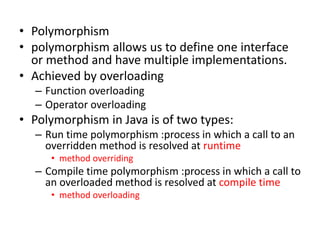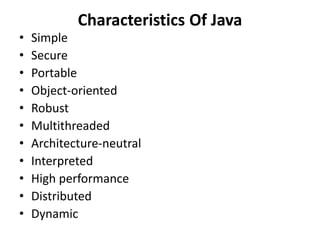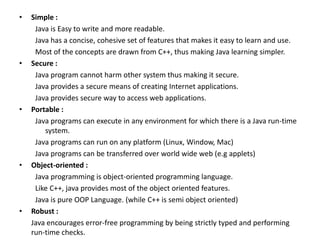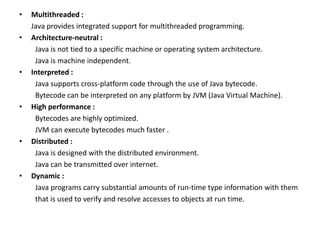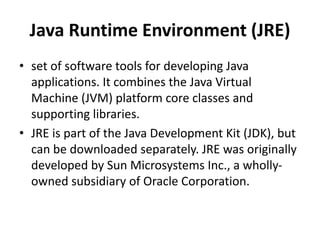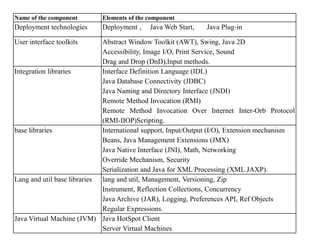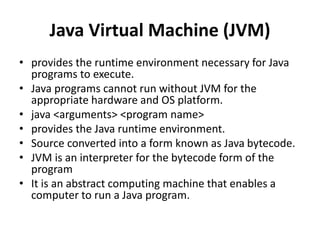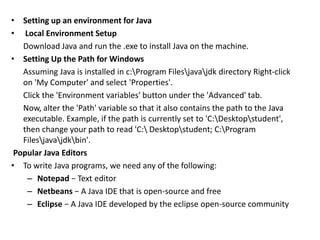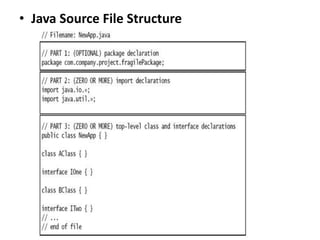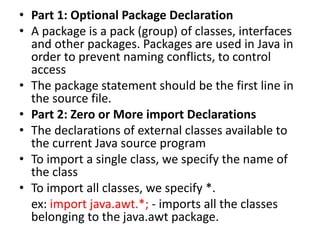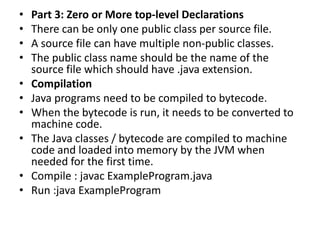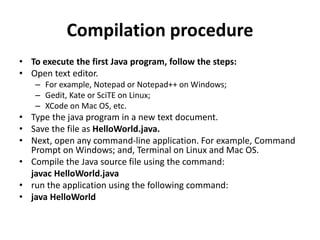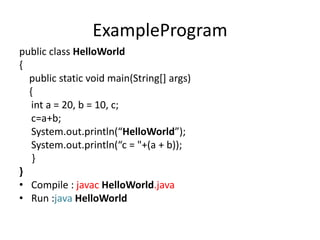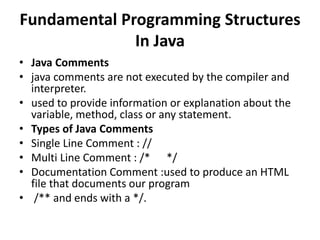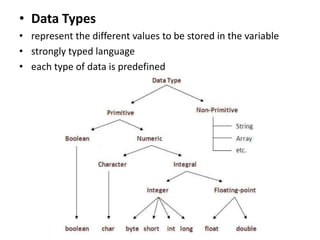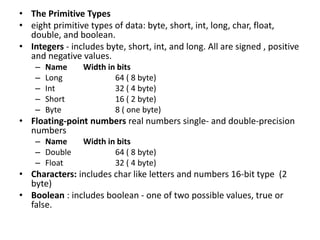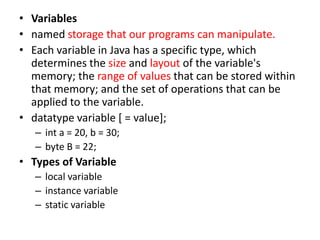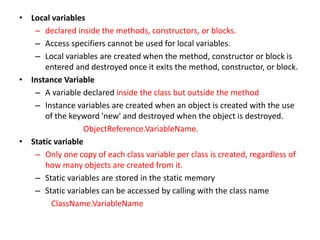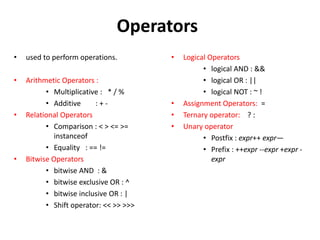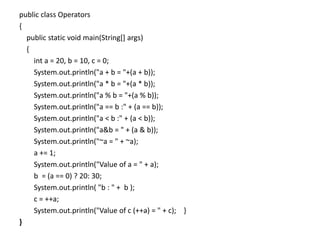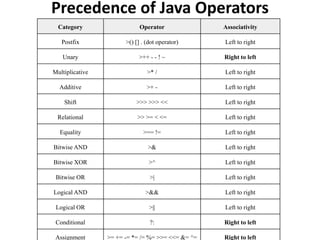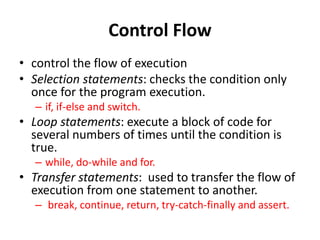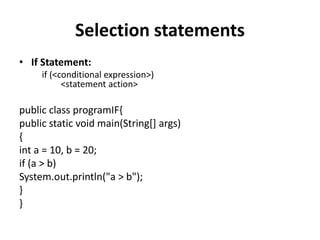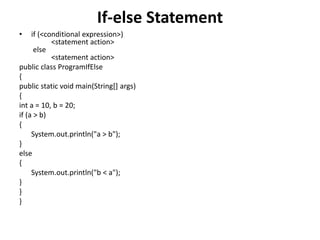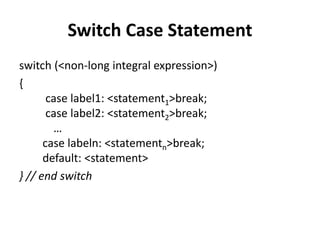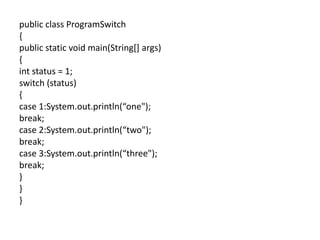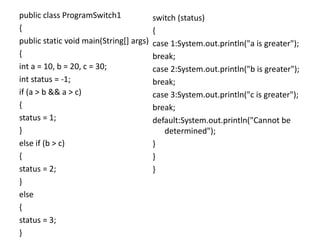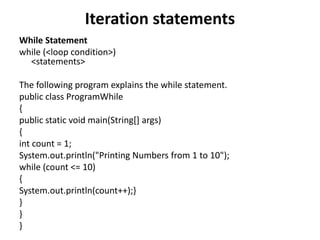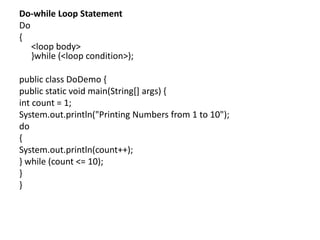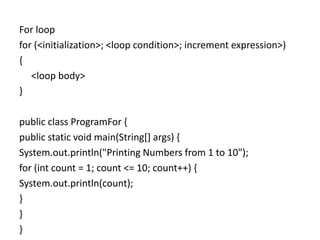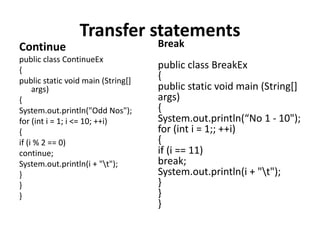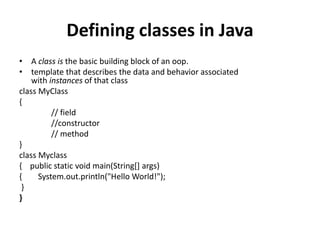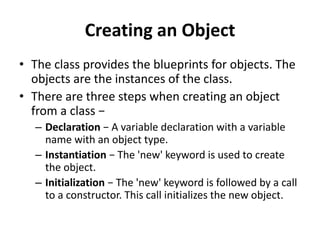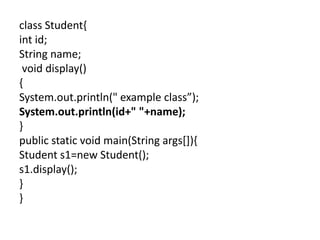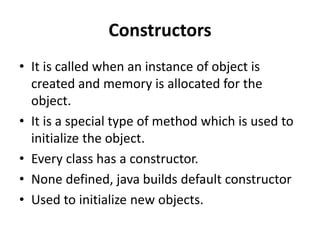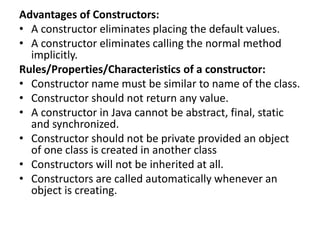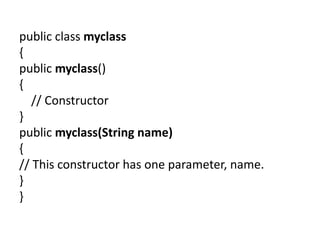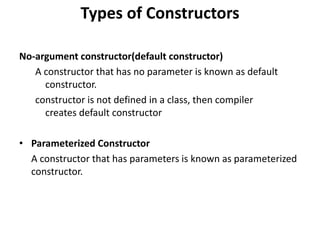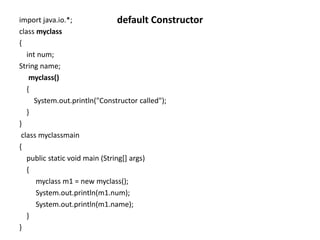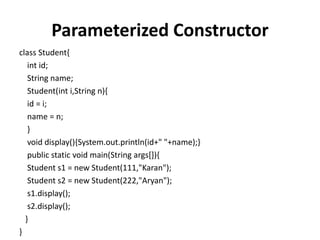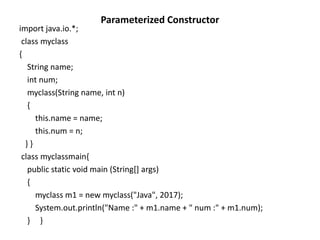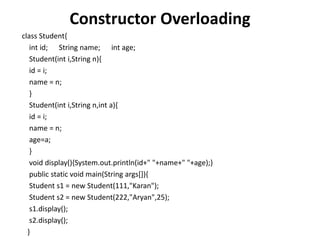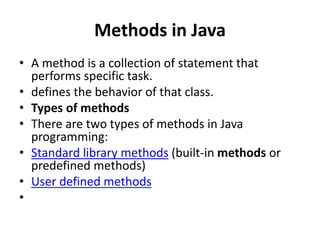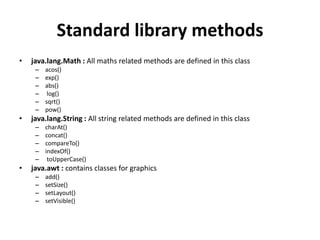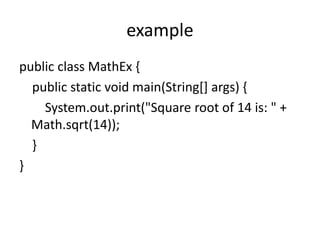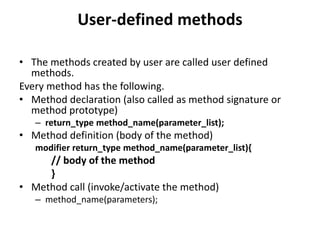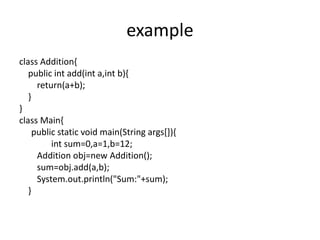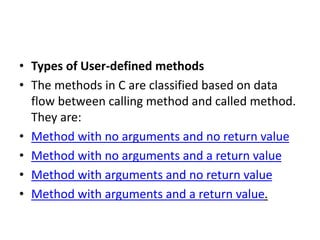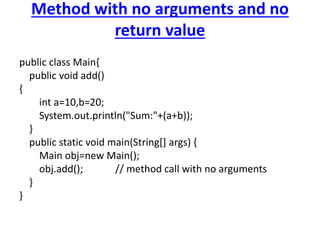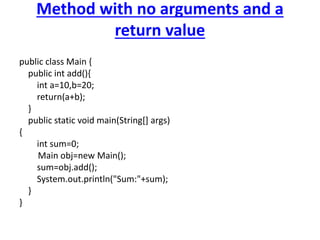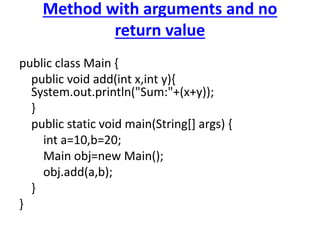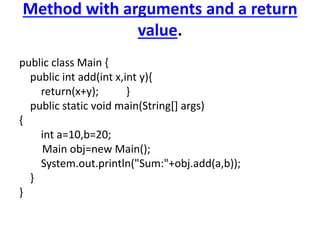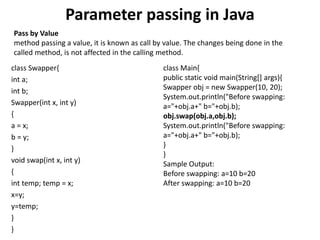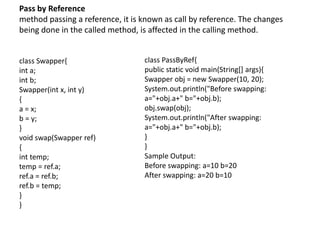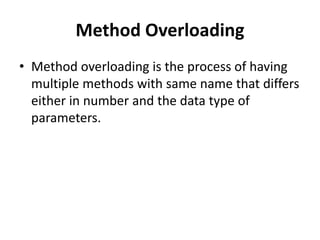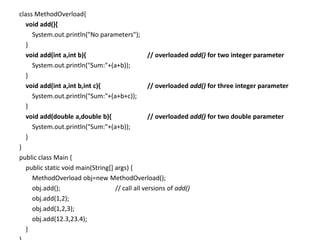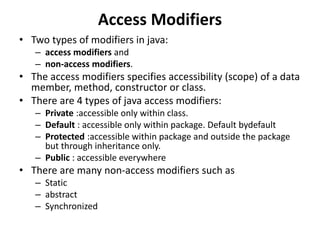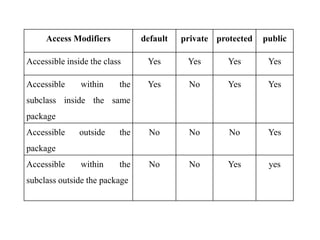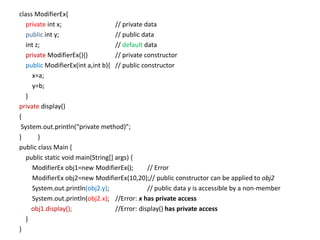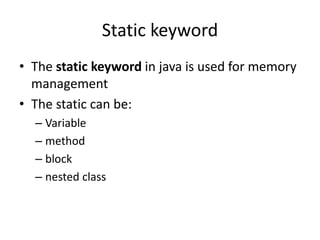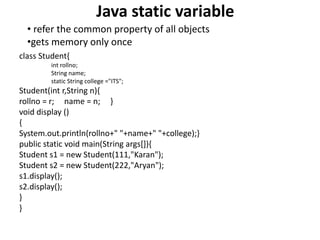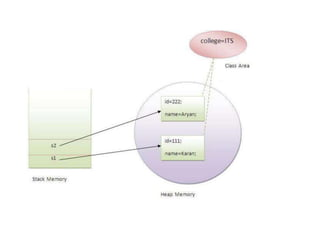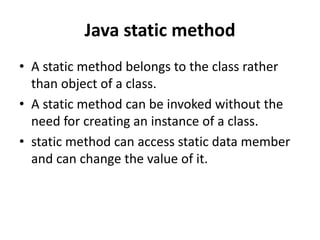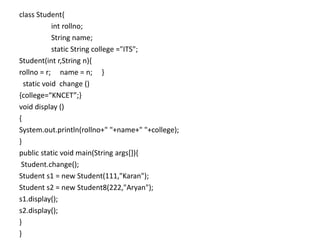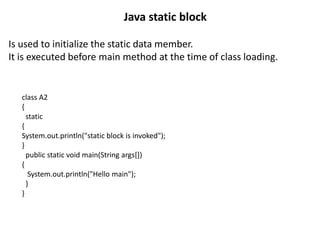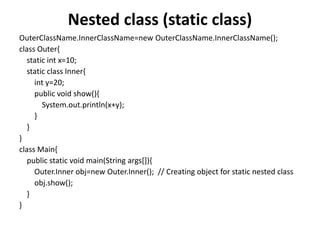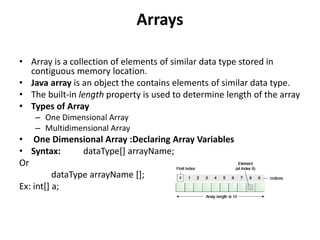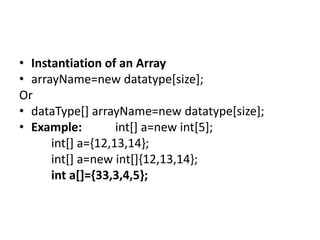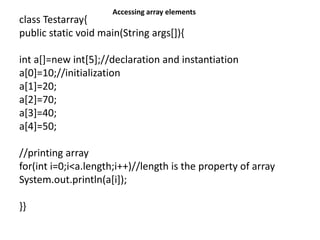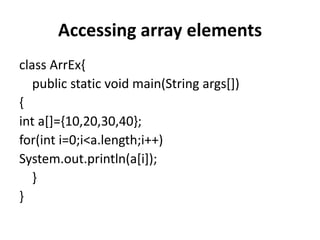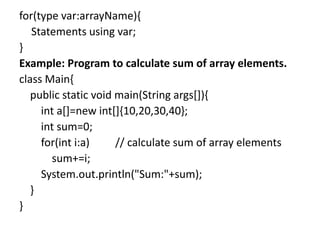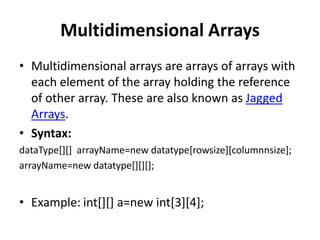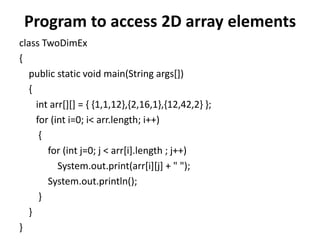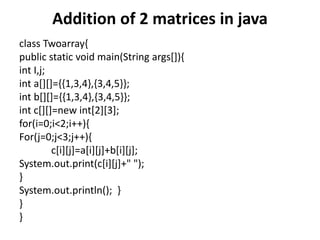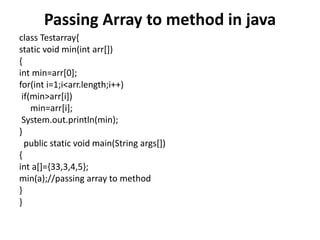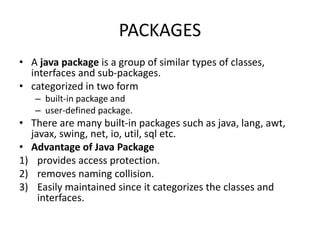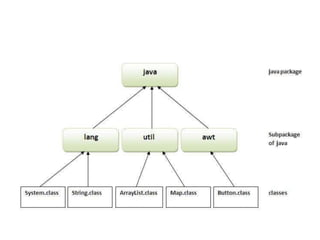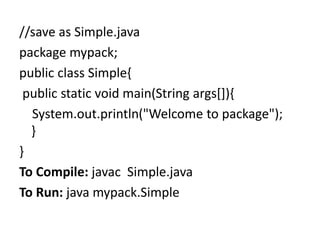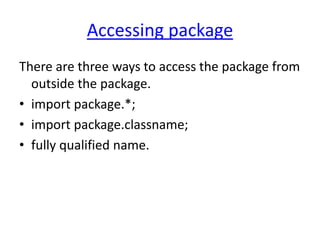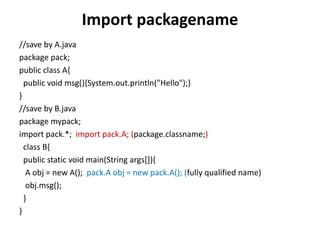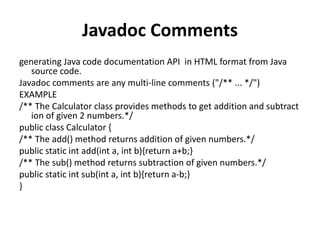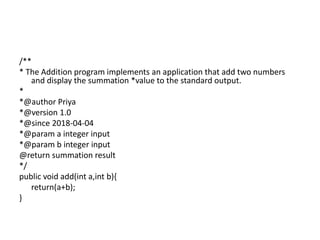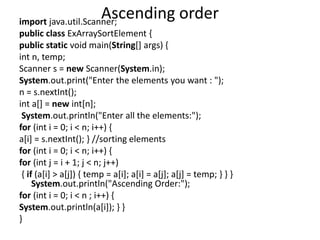1 von 89
Anzeige

### CS8392 OOP

1. CS8392-OBJECT ORIENTED PROGRAMMING L T P C 3 0 0 3 UNIT I - INTRODUCTION TO OOP AND JAVA FUNDAMENTALS 10 Object Oriented Programming - Abstraction – objects and classes - Encapsulation- Inheritance - Polymorphism- OOP in Java – Characteristics of Java – The Java Environment - Java Source File - Structure – Compilation. Fundamental Programming Structures in Java – Defining classes in Java – constructors, methods -access specifiers - static members -Comments, Data Types, Variables, Operators, Control Flow, Arrays , Packages - JavaDoc comments.
2. • Abstraction It handles complexity by hiding unnecessary details from the user Abstraction refers to the quality of dealing with ideas rather than events. It basically deals with hiding the details and showing the essential things to the user. • Objects and Classes Object − Objects have states and behaviors. Example: A dog has states - color, name, breed behaviors – wagging the tail, barking, eating. An object is an instance of a class. • Class − A class can be defined as a template or blueprint that describes the behavior/state that the object of its type support.
3. CLASS EXAMPLE public class Dog { String breed; int age; String color; void barking() { System.out.println(“Bark”); } } A class can contain any of the following variable types. • Local variables - Variables defined inside methods • Instance variables - variables within a class but outside any method • Class variables - variables declared within a class, outside any method.
4. • Encapsulation • Binding (or wrapping) code and data together into a single unit is known as encapsulation. Encapsulation is the mechanism that binds together code and the data it manipulates, and keeps both safe from outside interference and misuse. • Polymorphism • Polymorphism (from Greek, meaning “many forms”) is a feature that allows one interface to be used for a general class of actions.
5. OOP Concepts In Java • Object • Any entity that has state and behavior is known as an object. It can be either physical or logical. • Class & Instance • Collection of objects of the same kind is called class. It is a logical entity. • A Class is a 3-Compartment box . • Class Name • Static Attributes • Dynamic Behaviors Name (Identifier) Student Circle Variables (Static Attributes) name, gender, dept, marks radius, color Methods (Dynamic Behaviors) getDetails() calculateGrade() getRadius() printArea()
6. • Abstraction • Abstraction refers to the quality of dealing with ideas rather than events. It basically deals with hiding the details and showing the essential things to the user. • Abstract class: • If a class is declared abstract, it cannot be instantiated. So we cannot create an object of an abstract class. • Interface: • Interface in Java is a collection of abstract methods and static constants. In an interface, each method is public and abstract but it does not contain any constructor. Interface also helps to achieve multiple inheritance in Java. • Encapsulation • Binding (or wrapping) code and data together into a single unit is known as encapsulation. • Inheritance • A class which inherits the properties is known as ‘derived/child class’ whereas a class whose properties are inherited is known as ‘base/parent class’. • For eg, a child inherits the properties from his father.
7. • Polymorphism • polymorphism allows us to define one interface or method and have multiple implementations. • Achieved by overloading – Function overloading – Operator overloading • Polymorphism in Java is of two types: – Run time polymorphism :process in which a call to an overridden method is resolved at runtime • method overriding – Compile time polymorphism :process in which a call to an overloaded method is resolved at compile time • method overloading
8. Characteristics Of Java • Simple • Secure • Portable • Object-oriented • Robust • Multithreaded • Architecture-neutral • Interpreted • High performance • Distributed • Dynamic
9. • Simple : Java is Easy to write and more readable. Java has a concise, cohesive set of features that makes it easy to learn and use. Most of the concepts are drawn from C++, thus making Java learning simpler. • Secure : Java program cannot harm other system thus making it secure. Java provides a secure means of creating Internet applications. Java provides secure way to access web applications. • Portable : Java programs can execute in any environment for which there is a Java run-time system. Java programs can run on any platform (Linux, Window, Mac) Java programs can be transferred over world wide web (e.g applets) • Object-oriented : Java programming is object-oriented programming language. Like C++, java provides most of the object oriented features. Java is pure OOP Language. (while C++ is semi object oriented) • Robust : Java encourages error-free programming by being strictly typed and performing run-time checks.
10. • Multithreaded : Java provides integrated support for multithreaded programming. • Architecture-neutral : Java is not tied to a specific machine or operating system architecture. Java is machine independent. • Interpreted : Java supports cross-platform code through the use of Java bytecode. Bytecode can be interpreted on any platform by JVM (Java Virtual Machine). • High performance : Bytecodes are highly optimized. JVM can execute bytecodes much faster . • Distributed : Java is designed with the distributed environment. Java can be transmitted over internet. • Dynamic : Java programs carry substantial amounts of run-time type information with them that is used to verify and resolve accesses to objects at run time.
11. Java Runtime Environment (JRE) • set of software tools for developing Java applications. It combines the Java Virtual Machine (JVM) platform core classes and supporting libraries. • JRE is part of the Java Development Kit (JDK), but can be downloaded separately. JRE was originally developed by Sun Microsystems Inc., a wholly- owned subsidiary of Oracle Corporation.
12. Name of the component Elements of the component Deployment technologies Deployment , Java Web Start, Java Plug-in User interface toolkits Abstract Window Toolkit (AWT), Swing, Java 2D Accessibility, Image I/O, Print Service, Sound Drag and Drop (DnD),Input methods. Integration libraries Interface Definition Language (IDL) Java Database Connectivity (JDBC) Java Naming and Directory Interface (JNDI) Remote Method Invocation (RMI) Remote Method Invocation Over Internet Inter-Orb Protocol (RMI-IIOP)Scripting. base libraries International support, Input/Output (I/O), Extension mechanism Beans, Java Management Extensions (JMX) Java Native Interface (JNI), Math, Networking Override Mechanism, Security Serialization and Java for XML Processing (XML JAXP). Lang and util base libraries lang and util, Management, Versioning, Zip Instrument, Reflection Collections, Concurrency Java Archive (JAR), Logging, Preferences API, Ref Objects Regular Expressions. Java Virtual Machine (JVM) Java HotSpot Client Server Virtual Machines
13. Java Virtual Machine (JVM) • provides the runtime environment necessary for Java programs to execute. • Java programs cannot run without JVM for the appropriate hardware and OS platform. • java <arguments> <program name> • provides the Java runtime environment. • Source converted into a form known as Java bytecode. • JVM is an interpreter for the bytecode form of the program • It is an abstract computing machine that enables a computer to run a Java program.
14. • Setting up an environment for Java • Local Environment Setup Download Java and run the .exe to install Java on the machine. • Setting Up the Path for Windows Assuming Java is installed in c:Program Filesjavajdk directory Right-click on 'My Computer' and select 'Properties'. Click the 'Environment variables' button under the 'Advanced' tab. Now, alter the 'Path' variable so that it also contains the path to the Java executable. Example, if the path is currently set to 'C:Desktopstudent', then change your path to read 'C: Desktopstudent; C:Program Filesjavajdkbin'. Popular Java Editors • To write Java programs, we need any of the following: – Notepad − Text editor – Netbeans − A Java IDE that is open-source and free – Eclipse − A Java IDE developed by the eclipse open-source community
15. • Java Source File Structure
16. • Part 1: Optional Package Declaration • A package is a pack (group) of classes, interfaces and other packages. Packages are used in Java in order to prevent naming conflicts, to control access • The package statement should be the first line in the source file. • Part 2: Zero or More import Declarations • The declarations of external classes available to the current Java source program • To import a single class, we specify the name of the class • To import all classes, we specify *. ex: import java.awt.*; - imports all the classes belonging to the java.awt package.
17. • Part 3: Zero or More top-level Declarations • There can be only one public class per source file. • A source file can have multiple non-public classes. • The public class name should be the name of the source file which should have .java extension. • Compilation • Java programs need to be compiled to bytecode. • When the bytecode is run, it needs to be converted to machine code. • The Java classes / bytecode are compiled to machine code and loaded into memory by the JVM when needed for the first time. • Compile : javac ExampleProgram.java • Run :java ExampleProgram
18. Compilation procedure • To execute the first Java program, follow the steps: • Open text editor. – For example, Notepad or Notepad++ on Windows; – Gedit, Kate or SciTE on Linux; – XCode on Mac OS, etc. • Type the java program in a new text document. • Save the file as HelloWorld.java. • Next, open any command-line application. For example, Command Prompt on Windows; and, Terminal on Linux and Mac OS. • Compile the Java source file using the command: javac HelloWorld.java • run the application using the following command: • java HelloWorld
19. ExampleProgram public class HelloWorld { public static void main(String[] args) { int a = 20, b = 10, c; c=a+b; System.out.println(“HelloWorld”); System.out.println(“c = "+(a + b)); } } • Compile : javac HelloWorld.java • Run :java HelloWorld
20. Fundamental Programming Structures In Java • Java Comments • java comments are not executed by the compiler and interpreter. • used to provide information or explanation about the variable, method, class or any statement. • Types of Java Comments • Single Line Comment : // • Multi Line Comment : /* */ • Documentation Comment :used to produce an HTML file that documents our program • /** and ends with a */.
21. • Data Types • represent the different values to be stored in the variable • strongly typed language • each type of data is predefined
22. • The Primitive Types • eight primitive types of data: byte, short, int, long, char, float, double, and boolean. • Integers - includes byte, short, int, and long. All are signed , positive and negative values. – Name Width in bits – Long 64 ( 8 byte) – Int 32 ( 4 byte) – Short 16 ( 2 byte) – Byte 8 ( one byte) • Floating-point numbers real numbers single- and double-precision numbers – Name Width in bits – Double 64 ( 8 byte) – Float 32 ( 4 byte) • Characters: includes char like letters and numbers 16-bit type (2 byte) • Boolean : includes boolean - one of two possible values, true or false.
23. • Variables • named storage that our programs can manipulate. • Each variable in Java has a specific type, which determines the size and layout of the variable's memory; the range of values that can be stored within that memory; and the set of operations that can be applied to the variable. • datatype variable [ = value]; – int a = 20, b = 30; – byte B = 22; • Types of Variable – local variable – instance variable – static variable
24. • Local variables – declared inside the methods, constructors, or blocks. – Access specifiers cannot be used for local variables. – Local variables are created when the method, constructor or block is entered and destroyed once it exits the method, constructor, or block. • Instance Variable – A variable declared inside the class but outside the method – Instance variables are created when an object is created with the use of the keyword 'new' and destroyed when the object is destroyed. ObjectReference.VariableName. • Static variable – Only one copy of each class variable per class is created, regardless of how many objects are created from it. – Static variables are stored in the static memory – Static variables can be accessed by calling with the class name ClassName.VariableName
25. Operators • used to perform operations. • Arithmetic Operators : • Multiplicative : * / % • Additive : + - • Relational Operators • Comparison : < > <= >= instanceof • Equality : == != • Bitwise Operators • bitwise AND : & • bitwise exclusive OR : ^ • bitwise inclusive OR : | • Shift operator: << >> >>> • Logical Operators • logical AND : && • logical OR : || • logical NOT : ~ ! • Assignment Operators: = • Ternary operator: ? : • Unary operator • Postfix : expr++ expr— • Prefix : ++expr --expr +expr - expr
26. public class Operators { public static void main(String[] args) { int a = 20, b = 10, c = 0; System.out.println("a + b = "+(a + b)); System.out.println("a * b = "+(a * b)); System.out.println("a % b = "+(a % b)); System.out.println("a == b :" + (a == b)); System.out.println("a < b :" + (a < b)); System.out.println("a&b = " + (a & b)); System.out.println("~a = " + ~a); a += 1; System.out.println("Value of a = " + a); b = (a == 0) ? 20: 30; System.out.println( "b : " + b ); c = ++a; System.out.println("Value of c (++a) = " + c); } }
27. Precedence of Java Operators Category Operator Associativity Postfix >() [] . (dot operator) Left to right Unary >++ - - ! ~ Right to left Multiplicative >* / Left to right Additive >+ - Left to right Shift >>> >>> << Left to right Relational >> >= < <= Left to right Equality >== != Left to right Bitwise AND >& Left to right Bitwise XOR >^ Left to right Bitwise OR >| Left to right Logical AND >&& Left to right Logical OR >|| Left to right Conditional ?: Right to left Assignment >= += -= *= /= %= >>= <<= &= ^= Right to left
28. Control Flow • control the flow of execution • Selection statements: checks the condition only once for the program execution. – if, if-else and switch. • Loop statements: execute a block of code for several numbers of times until the condition is true. – while, do-while and for. • Transfer statements: used to transfer the flow of execution from one statement to another. – break, continue, return, try-catch-finally and assert.
29. Selection statements • If Statement: if (<conditional expression>) <statement action> public class programIF{ public static void main(String[] args) { int a = 10, b = 20; if (a > b) System.out.println("a > b"); } }
30. If-else Statement • if (<conditional expression>) <statement action> else <statement action> public class ProgramIfElse { public static void main(String[] args) { int a = 10, b = 20; if (a > b) { System.out.println("a > b"); } else { System.out.println("b < a"); } } }
31. Switch Case Statement switch (<non-long integral expression>) { case label1: <statement1>break; case label2: <statement2>break; … case labeln: <statementn>break; default: <statement> } // end switch
32. public class ProgramSwitch { public static void main(String[] args) { int status = 1; switch (status) { case 1:System.out.println(“one"); break; case 2:System.out.println(“two"); break; case 3:System.out.println(“three"); break; } } }
33. public class ProgramSwitch1 { public static void main(String[] args) { int a = 10, b = 20, c = 30; int status = -1; if (a > b && a > c) { status = 1; } else if (b > c) { status = 2; } else { status = 3; } switch (status) { case 1:System.out.println("a is greater"); break; case 2:System.out.println("b is greater"); break; case 3:System.out.println("c is greater"); break; default:System.out.println("Cannot be determined"); } } }
34. Iteration statements While Statement while (<loop condition>) <statements> The following program explains the while statement. public class ProgramWhile { public static void main(String[] args) { int count = 1; System.out.println("Printing Numbers from 1 to 10"); while (count <= 10) { System.out.println(count++);} } } }
35. Do-while Loop Statement Do { <loop body> }while (<loop condition>); public class DoDemo { public static void main(String[] args) { int count = 1; System.out.println("Printing Numbers from 1 to 10"); do { System.out.println(count++); } while (count <= 10); } }
36. For loop for (<initialization>; <loop condition>; increment expression>) { <loop body> } public class ProgramFor { public static void main(String[] args) { System.out.println("Printing Numbers from 1 to 10"); for (int count = 1; count <= 10; count++) { System.out.println(count); } } }
37. Transfer statements Continue public class ContinueEx { public static void main (String[] args) { System.out.println("Odd Nos"); for (int i = 1; i <= 10; ++i) { if (i % 2 == 0) continue; System.out.println(i + "t"); } } } Break public class BreakEx { public static void main (String[] args) { System.out.println(“No 1 - 10"); for (int i = 1;; ++i) { if (i == 11) break; System.out.println(i + "t"); } } }
38. Defining classes in Java • A class is the basic building block of an oop. • template that describes the data and behavior associated with instances of that class class MyClass { // field //constructor // method } class Myclass { public static void main(String[] args) { System.out.println("Hello World!"); } }
39. Creating an Object • The class provides the blueprints for objects. The objects are the instances of the class. • There are three steps when creating an object from a class − – Declaration − A variable declaration with a variable name with an object type. – Instantiation − The 'new' keyword is used to create the object. – Initialization − The 'new' keyword is followed by a call to a constructor. This call initializes the new object.
40. class Student{ int id; String name; void display() { System.out.println(" example class”); System.out.println(id+" "+name); } public static void main(String args[]){ Student s1=new Student(); s1.display(); } }
41. Constructors • It is called when an instance of object is created and memory is allocated for the object. • It is a special type of method which is used to initialize the object. • Every class has a constructor. • None defined, java builds default constructor • Used to initialize new objects.
42. Advantages of Constructors: • A constructor eliminates placing the default values. • A constructor eliminates calling the normal method implicitly. Rules/Properties/Characteristics of a constructor: • Constructor name must be similar to name of the class. • Constructor should not return any value. • A constructor in Java cannot be abstract, final, static and synchronized. • Constructor should not be private provided an object of one class is created in another class • Constructors will not be inherited at all. • Constructors are called automatically whenever an object is creating.
43. public class myclass { public myclass() { // Constructor } public myclass(String name) { // This constructor has one parameter, name. } }
44. Types of Constructors No-argument constructor(default constructor) A constructor that has no parameter is known as default constructor. constructor is not defined in a class, then compiler creates default constructor • Parameterized Constructor A constructor that has parameters is known as parameterized constructor.
45. import java.io.*; class myclass { int num; String name; myclass() { System.out.println("Constructor called"); } } class myclassmain { public static void main (String[] args) { myclass m1 = new myclass(); System.out.println(m1.num); System.out.println(m1.name); } } default Constructor
46. Parameterized Constructor class Student{ int id; String name; Student(int i,String n){ id = i; name = n; } void display(){System.out.println(id+" "+name);} public static void main(String args[]){ Student s1 = new Student(111,"Karan"); Student s2 = new Student(222,"Aryan"); s1.display(); s2.display(); } }
47. Parameterized Constructor import java.io.*; class myclass { String name; int num; myclass(String name, int n) { this.name = name; this.num = n; } } class myclassmain{ public static void main (String[] args) { myclass m1 = new myclass("Java", 2017); System.out.println("Name :" + m1.name + " num :" + m1.num); } }
48. Constructor Overloading class Student{ int id; String name; int age; Student(int i,String n){ id = i; name = n; } Student(int i,String n,int a){ id = i; name = n; age=a; } void display(){System.out.println(id+" "+name+" "+age);} public static void main(String args[]){ Student s1 = new Student(111,"Karan"); Student s2 = new Student(222,"Aryan",25); s1.display(); s2.display(); }
49. Methods in Java • A method is a collection of statement that performs specific task. • defines the behavior of that class. • Types of methods • There are two types of methods in Java programming: • Standard library methods (built-in methods or predefined methods) • User defined methods •
50. Standard library methods • java.lang.Math : All maths related methods are defined in this class – acos() – exp() – abs() – log() – sqrt() – pow() • java.lang.String : All string related methods are defined in this class – charAt() – concat() – compareTo() – indexOf() – toUpperCase() • java.awt : contains classes for graphics – add() – setSize() – setLayout() – setVisible()
51. example public class MathEx { public static void main(String[] args) { System.out.print("Square root of 14 is: " + Math.sqrt(14)); } }
52. User-defined methods • The methods created by user are called user defined methods. Every method has the following. • Method declaration (also called as method signature or method prototype) – return_type method_name(parameter_list); • Method definition (body of the method) modifier return_type method_name(parameter_list){ // body of the method } • Method call (invoke/activate the method) – method_name(parameters);
54. • Types of User-defined methods • The methods in C are classified based on data flow between calling method and called method. They are: • Method with no arguments and no return value • Method with no arguments and a return value • Method with arguments and no return value • Method with arguments and a return value.
55. Method with no arguments and no return value public class Main{ public void add() { int a=10,b=20; System.out.println("Sum:"+(a+b)); } public static void main(String[] args) { Main obj=new Main(); obj.add(); // method call with no arguments } }
56. Method with no arguments and a return value public class Main { public int add(){ int a=10,b=20; return(a+b); } public static void main(String[] args) { int sum=0; Main obj=new Main(); sum=obj.add(); System.out.println("Sum:"+sum); } }
57. Method with arguments and no return value public class Main { public void add(int x,int y){ System.out.println("Sum:"+(x+y)); } public static void main(String[] args) { int a=10,b=20; Main obj=new Main(); obj.add(a,b); } }
58. Method with arguments and a return value. public class Main { public int add(int x,int y){ return(x+y); } public static void main(String[] args) { int a=10,b=20; Main obj=new Main(); System.out.println("Sum:"+obj.add(a,b)); } }
59. Parameter passing in Java class Swapper{ int a; int b; Swapper(int x, int y) { a = x; b = y; } void swap(int x, int y) { int temp; temp = x; x=y; y=temp; } } class Main{ public static void main(String[] args){ Swapper obj = new Swapper(10, 20); System.out.println("Before swapping: a="+obj.a+" b="+obj.b); obj.swap(obj.a,obj.b); System.out.println("Before swapping: a="+obj.a+" b="+obj.b); } } Sample Output: Before swapping: a=10 b=20 After swapping: a=10 b=20 Pass by Value method passing a value, it is known as call by value. The changes being done in the called method, is not affected in the calling method.
60. Pass by Reference method passing a reference, it is known as call by reference. The changes being done in the called method, is affected in the calling method. class Swapper{ int a; int b; Swapper(int x, int y) { a = x; b = y; } void swap(Swapper ref) { int temp; temp = ref.a; ref.a = ref.b; ref.b = temp; } } class PassByRef{ public static void main(String[] args){ Swapper obj = new Swapper(10, 20); System.out.println("Before swapping: a="+obj.a+" b="+obj.b); obj.swap(obj); System.out.println("After swapping: a="+obj.a+" b="+obj.b); } } Sample Output: Before swapping: a=10 b=20 After swapping: a=20 b=10
61. Method Overloading • Method overloading is the process of having multiple methods with same name that differs either in number and the data type of parameters.
63. Access Modifiers • Two types of modifiers in java: – access modifiers and – non-access modifiers. • The access modifiers specifies accessibility (scope) of a data member, method, constructor or class. • There are 4 types of java access modifiers: – Private :accessible only within class. – Default : accessible only within package. Default bydefault – Protected :accessible within package and outside the package but through inheritance only. – Public : accessible everywhere • There are many non-access modifiers such as – Static – abstract – Synchronized
64. Access Modifiers default private protected public Accessible inside the class Yes Yes Yes Yes Accessible within the subclass inside the same package Yes No Yes Yes Accessible outside the package No No No Yes Accessible within the subclass outside the package No No Yes yes
65. class ModifierEx{ private int x; // private data public int y; // public data int z; // default data private ModifierEx(){} // private constructor public ModifierEx(int a,int b){ // public constructor x=a; y=b; } private display() { System.out.println(“private method)”; } } public class Main { public static void main(String[] args) { ModifierEx obj1=new ModifierEx(); // Error ModifierEx obj2=new ModifierEx(10,20);// public constructor can be applied to obj2 System.out.println(obj2.y); // public data y is accessible by a non-member System.out.println(obj2.x); //Error: x has private access obj1.display(); //Error: display() has private access } }
66. Static keyword • The static keyword in java is used for memory management • The static can be: – Variable – method – block – nested class
67. Java static variable class Student{ int rollno; String name; static String college ="ITS"; Student(int r,String n){ rollno = r; name = n; } void display () { System.out.println(rollno+" "+name+" "+college);} public static void main(String args[]){ Student s1 = new Student(111,"Karan"); Student s2 = new Student(222,"Aryan"); s1.display(); s2.display(); } } • refer the common property of all objects •gets memory only once
68. Java static method • A static method belongs to the class rather than object of a class. • A static method can be invoked without the need for creating an instance of a class. • static method can access static data member and can change the value of it.
69. class Student{ int rollno; String name; static String college ="ITS"; Student(int r,String n){ rollno = r; name = n; } static void change () {college=“KNCET”;} void display () { System.out.println(rollno+" "+name+" "+college); } public static void main(String args[]){ Student.change(); Student s1 = new Student(111,"Karan"); Student s2 = new Student8(222,"Aryan"); s1.display(); s2.display(); } }
70. Is used to initialize the static data member. It is executed before main method at the time of class loading. Java static block class A2 { static { System.out.println("static block is invoked"); } public static void main(String args[]) { System.out.println("Hello main"); } }
71. Nested class (static class) OuterClassName.InnerClassName=new OuterClassName.InnerClassName(); class Outer{ static int x=10; static class Inner{ int y=20; public void show(){ System.out.println(x+y); } } } class Main{ public static void main(String args[]){ Outer.Inner obj=new Outer.Inner(); // Creating object for static nested class obj.show(); } }
72. Arrays • Array is a collection of elements of similar data type stored in contiguous memory location. • Java array is an object the contains elements of similar data type. • The built-in length property is used to determine length of the array • Types of Array – One Dimensional Array – Multidimensional Array • One Dimensional Array :Declaring Array Variables • Syntax: dataType[] arrayName; Or dataType arrayName []; Ex: int[] a;
73. • Instantiation of an Array • arrayName=new datatype[size]; Or • dataType[] arrayName=new datatype[size]; • Example: int[] a=new int; int[] a={12,13,14}; int[] a=new int[]{12,13,14}; int a[]={33,3,4,5};
74. class Testarray{ public static void main(String args[]){ int a[]=new int;//declaration and instantiation a=10;//initialization a=20; a=70; a=40; a=50; //printing array for(int i=0;i<a.length;i++)//length is the property of array System.out.println(a[i]); }} Accessing array elements
75. Accessing array elements class ArrEx{ public static void main(String args[]) { int a[]={10,20,30,40}; for(int i=0;i<a.length;i++) System.out.println(a[i]); } }
76. for(type var:arrayName){ Statements using var; } Example: Program to calculate sum of array elements. class Main{ public static void main(String args[]){ int a[]=new int[]{10,20,30,40}; int sum=0; for(int i:a) // calculate sum of array elements sum+=i; System.out.println("Sum:"+sum); } }
77. Multidimensional Arrays • Multidimensional arrays are arrays of arrays with each element of the array holding the reference of other array. These are also known as Jagged Arrays. • Syntax: dataType[][] arrayName=new datatype[rowsize][columnnsize]; arrayName=new datatype[][][]; • Example: int[][] a=new int;
78. Program to access 2D array elements class TwoDimEx { public static void main(String args[]) { int arr[][] = { {1,1,12},{2,16,1},{12,42,2} }; for (int i=0; i< arr.length; i++) { for (int j=0; j < arr[i].length ; j++) System.out.print(arr[i][j] + " "); System.out.println(); } } }
79. Addition of 2 matrices in java class Twoarray{ public static void main(String args[]){ int I,j; int a[][]={{1,3,4},{3,4,5}}; int b[][]={{1,3,4},{3,4,5}}; int c[][]=new int; for(i=0;i<2;i++){ For(j=0;j<3;j++){ c[i][j]=a[i][j]+b[i][j]; System.out.print(c[i][j]+" "); } System.out.println(); } } }
80. Passing Array to method in java class Testarray{ static void min(int arr[]) { int min=arr; for(int i=1;i<arr.length;i++) if(min>arr[i]) min=arr[i]; System.out.println(min); } public static void main(String args[]) { int a[]={33,3,4,5}; min(a);//passing array to method } }
81. PACKAGES • A java package is a group of similar types of classes, interfaces and sub-packages. • categorized in two form – built-in package and – user-defined package. • There are many built-in packages such as java, lang, awt, javax, swing, net, io, util, sql etc. • Advantage of Java Package 1) provides access protection. 2) removes naming collision. 3) Easily maintained since it categorizes the classes and interfaces.
82. //save as Simple.java package mypack; public class Simple{ public static void main(String args[]){ System.out.println("Welcome to package"); } } To Compile: javac Simple.java To Run: java mypack.Simple
83. Accessing package There are three ways to access the package from outside the package. • import package.*; • import package.classname; • fully qualified name.
84. Import packagename //save by A.java package pack; public class A{ public void msg(){System.out.println("Hello");} } //save by B.java package mypack; import pack.*; import pack.A; (package.classname;) class B{ public static void main(String args[]){ A obj = new A(); pack.A obj = new pack.A(); (fully qualified name) obj.msg(); } }
85. Javadoc Comments generating Java code documentation API in HTML format from Java source code. Javadoc comments are any multi-line comments ("/** ... */") EXAMPLE /** The Calculator class provides methods to get addition and subtract ion of given 2 numbers.*/ public class Calculator { /** The add() method returns addition of given numbers.*/ public static int add(int a, int b){return a+b;} /** The sub() method returns subtraction of given numbers.*/ public static int sub(int a, int b){return a-b;} }
86. /** * The Addition program implements an application that add two numbers and display the summation *value to the standard output. * *@author Priya *@version 1.0 *@since 2018-04-04 *@param a integer input *@param b integer input @return summation result */ public void add(int a,int b){ return(a+b); }
87. Ascending orderimport java.util.Scanner; public class ExArraySortElement { public static void main(String[] args) { int n, temp; Scanner s = new Scanner(System.in); System.out.print("Enter the elements you want : "); n = s.nextInt(); int a[] = new int[n]; System.out.println("Enter all the elements:"); for (int i = 0; i < n; i++) { a[i] = s.nextInt(); } //sorting elements for (int i = 0; i < n; i++) { for (int j = i + 1; j < n; j++) { if (a[i] > a[j]) { temp = a[i]; a[i] = a[j]; a[j] = temp; } } } System.out.println("Ascending Order:"); for (int i = 0; i < n ; i++) { System.out.println(a[i]); } } }
Anzeige